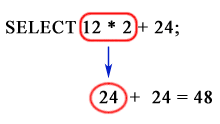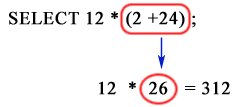# Oracle Unary and Binary Operators

## Introduction

The two general classes of operators are :

unary : A unary operator is an operator that operates on only one operand. Here is the format :

`operator operand`

Example: +2460, -300

binary : An operator is referred to as binary if it operates on two operands. Here is the format :

`operand1 operator operand2`

Example: 1254+2564, 2460-300

Other operators with special formats accept more than two operands. If an operator is given a null operand, then the result is always null. The concatenation (||) does not follow this rule.

Operator Precedence

Precedence is the order in which database evaluates different operators in the same expression. When evaluating an expression containing multiple operators (e.g. +, -, /), operator precedence evaluates operators with higher precedence before evaluating those with lower precedence. Operator precedence evaluates operators with equal precedence from left to right within an expression.
If there are parentheses within the expression then it evaluated first and the rest part which are outside the parentheses are evaluated next. Oracle evaluates operators with equal precedence from left to right within an expression.

The following table lists the levels of precedence among Oracle operators from high to low.

Operators Operation
+, - (as unary operators)
PRIOR
CONNECT_BY_ROOT
Identity
negation
location in hierarchy
*
/
Multiplication
division
+
- (as binary operators)
||
Subtraction
Concatenation

Examples: Binary Operators

+ operator:

You can use the + operator to add two numeric values. Here is an example:

``````SQL> SELECT 125 + 34 FROM DUAL;
```
```

Sample Output:

``` 125+34
----------
159```

- operator:

You can use the - operator subtract two numeric values. Here is an example:

```SQL> SELECT 125 - 34 FROM DUAL;
125-34
----------
91
```

* operator:

You can use the * operator to multiply one number to another. Here is an example:

```SQL> SELECT 125 * 34 FROM DUAL;
125*34
----------
4250
```

/ operator:

You can use the / operator to divide one number from another. Here is an example:

```SQL> SELECT 125/34 FROM DUAL;
125/34
------------
3.67647059```

Example - 1: Oracle Operators Precedence

In the following expression, multiplication has a higher precedence than addition, so Oracle first multiplies 20 by 30 and then adds the result to 10.

```SQL> SELECT 10+20*30 FROM DUAL;
10+20*30
----------
610```

You can use parentheses in an expression to override operator precedence. Oracle evaluates expressions inside parentheses before evaluating those outside.

The following expression in Oracle query return different results :

```SQL> SELECT 12*2+24 FROM DUAL;
12*2+24
----------
48
``````SQL> SELECT 12*(2+24) FROM DUAL;
12*(2+24)
----------
312
```Previous: Literals
Next: Arithmetic Operators

﻿